#### Statistics I - Question paper 2076

##### Other question papers
2075 2076 2078 Model QuestionTribhuwan University
Institute of Science and Technology
2076

Bachelor Level / second-semester / Science
Computer Science and Information Technology(STA164)
(Statistics I)
Candidates are required to give their answers in their own words as far as practicable.
The figures in the margin indicate full marks.
Full Marks: 60
Pass Marks: 24
Time: 3 hours

Group A

Attempt any two questions:(2 x 10 = 20)

1. What are the roles of measure of dispersion in descriptive statistics? Following table gives the frequency distribution of thickness of computer chips (in nanometer) manufactured by two companies.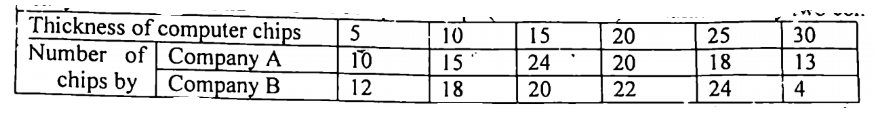Which company may be considered more consistent in terms of thickness of computer chips? Apply appropriate descriptive statistics.

10 Marks

2. A study was done to study the effect of ambient temperature on the electric power consumed by a chemical plant. Following table gives the data which are collected from an experimental pilot plant.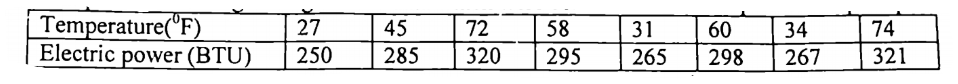(i) Identify which one is response variable, and fit a simple regression line, assuming that the relationship between them is linear.

(ii) Interpret the regression coefficient with reference to your problem.

(iii) Obtain coefficient of determination, and interpret this.

(iv) Based on the fitted model in (a), predict the power consumption for an ambient temperature of 650F.

10 Marks

3. (a) Define Normal distribution. What are the main characteristics of a Normal distribution?

(b) What do you mean by probability density function? Write down its properties.

10 Marks

Group B

Attempt any Eight questions:

4. The following table gives the installation time (in minutes) for hardware on 50 different computers.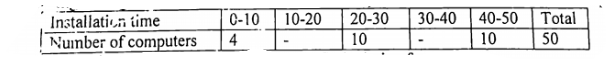If the average installation time is 30.2 minutes, find missing frequencies.

5 Marks

5. The length of power failure in minute are recorded in the following table.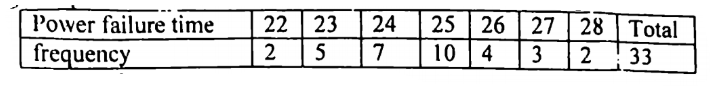Find Q3, D2 and P40 and interpret the results

5 Marks

6. A manufacturing company employs three analytical plans for the design and development of a particular product. For cost reasons, all three are used at varying times. In facts, plan 1,2 and 3 are used for 30%, 20% and 50% of the products respectively. The defect rate in different procedures is as follows: P(D/P1) = 0.01, P(D/P2) = 0.03, P(D/P3)= 0.02, where P(D/PJ) is the probability of a defective product, given plan j. If a random product was observed and found to be defective, which plan was most likely used and thus responsible?

5 Marks

7. The random variable X has following probability distribution.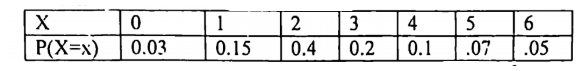Find (i) E(X) and var(X) (ii) Calculate E(Y) if Y = 3X + 5.

5 Marks

8. If two random variables have the joint probability density function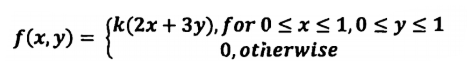Find (i) constant k (ii) Conditional probability density function of x given Y (iii) Identify whether X and Y are independent.

5 Marks

9. A large chain retailer purchases a certain kind of electronic device from a manufacturer. The manufacturer indicates that the defective rate of the device is 15%. The inspector randomly picks 10 items from a shipment. What is the probability that there will be at least one defective item among these 10?

5 Marks

10. Messages arrive at an electronic message center at random times, with an average of 9 messages per hour.

a) What is the probability of receiving at least four messages during the next hour?

b) What is the probability of receiving at most three messages during the next hour?

5 Marks

11. Following data represent the preference of 10 students studying B.Sc.(CSIT) towards two brands of computers namely DELL and HP.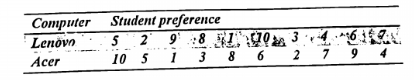Apply appropriate statistical tool to measure whether the brand preference is correlated. Also interpret your result.

5 Marks

12. What do you mean by measurement scale? Describe the different types of measurement scales used in statistics.

5 Marks

13. What is sampling? Discuss various probability sampling techniques with merits and demerits.

5 Marks